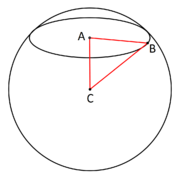# Circle of a sphere

Circle of a sphereSmall circle of a sphere.BC2 = AB2 + AC2, where C is the center of the sphere, A is the center of the small circle, and B is a point in the boundary of the small circle. Therefore, knowing the radius of the sphere, and the distance from the plane of the small circle to C, the radius of the small circle can be determined using the Pythagorean theorem.

A circle of a sphere is a circle defined as the intersection of a sphere and a plane. If the plane contains the center of the sphere then the circle is called a great circle, otherwise it is a small circle. Circles of a sphere have radius less than or equal to the radius sphere, with equality when the circle is a great circle.

In the geographic coordinate system on a globe, the parallels of latitude are such circles, with the Equator the only great circle. By contrast, all meridians of longitude, paired with their opposite meridian in the other hemisphere, form great circles.

The diameter of the sphere when passes though the center of the circle is called its axis and the endpoints of this diameter are called its poles. A circle of a sphere can also be defined as the set of points at a given angular distance from a given pole.

## Geometric proof

That the intersection of a sphere and a plane is, in fact, a circle can be seen as follows. Let the S be a sphere with center O, P a plane which intersects S. Draw OE perpendicular to P and meeting P at E. , Let A and B be any two points in the intersection. Then AOE and BOE are right triangles with a common side, OE, and hypotenuses AO and BO, equal. Therefore the remaining sides AE and BE are equal. This proves that all points in the intersection are the same distance from the point E in the plane P, in other words all points in the intersection lie on a circle with center E. Note that OE is the axis of the circle.

As a corollary, on a sphere there is exactly one circle that can be drawn though three given points.

The proof can be extended to show that the points on a circle are all a common angular distance from one of its poles.